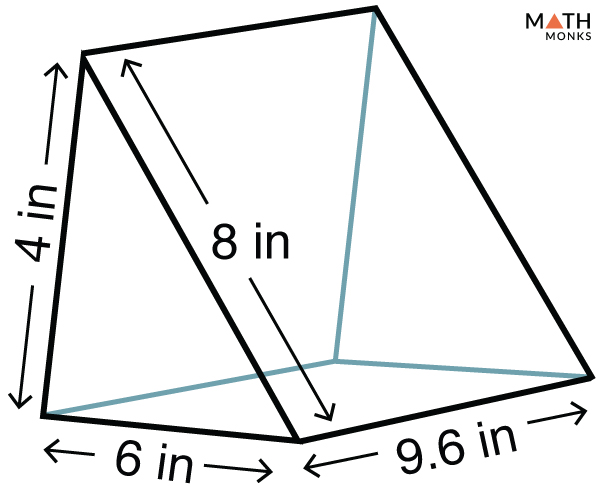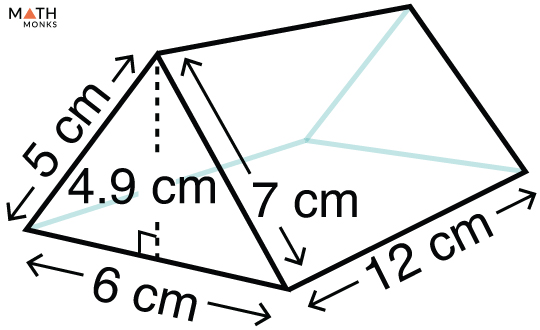# Surface Area of a Triangular Prism

The surface area of a triangular prism is the entire space occupied by its outermost layers (or faces). It is expressed in square units such as m2, cm2, mm2, and in2.

## Formulas

Like all 3-dimensional shapes, 2 types of surface areas can be calculated for a triangular prism.

### Lateral Surface Area

The lateral surface area (LSA) of a triangular prism is only the sum of the areas of all its faces except the bases. The formula to calculate the total and lateral surface area of a triangular prism is given below:

Let us solve an example to understand the concept better.Find the lateral surface area of a triangular prism given in the figure.

Solution:

As we know,
Lateral Surface Area (LSA) = (s1 + s2 + s3) × l, here s1 = 4 in, s2 = 6 in,  s3 = 8 in, l = 9.6 in
= 18 × 9.6
= 172.8 in2

### Total Surface Area

The total surface area (TSA) of a triangular prism is the sum of the lateral surface area and twice the base area. The formula to calculate the TSA of a triangular prism is given below:Find the total surface area of a triangular prism given in the figure.